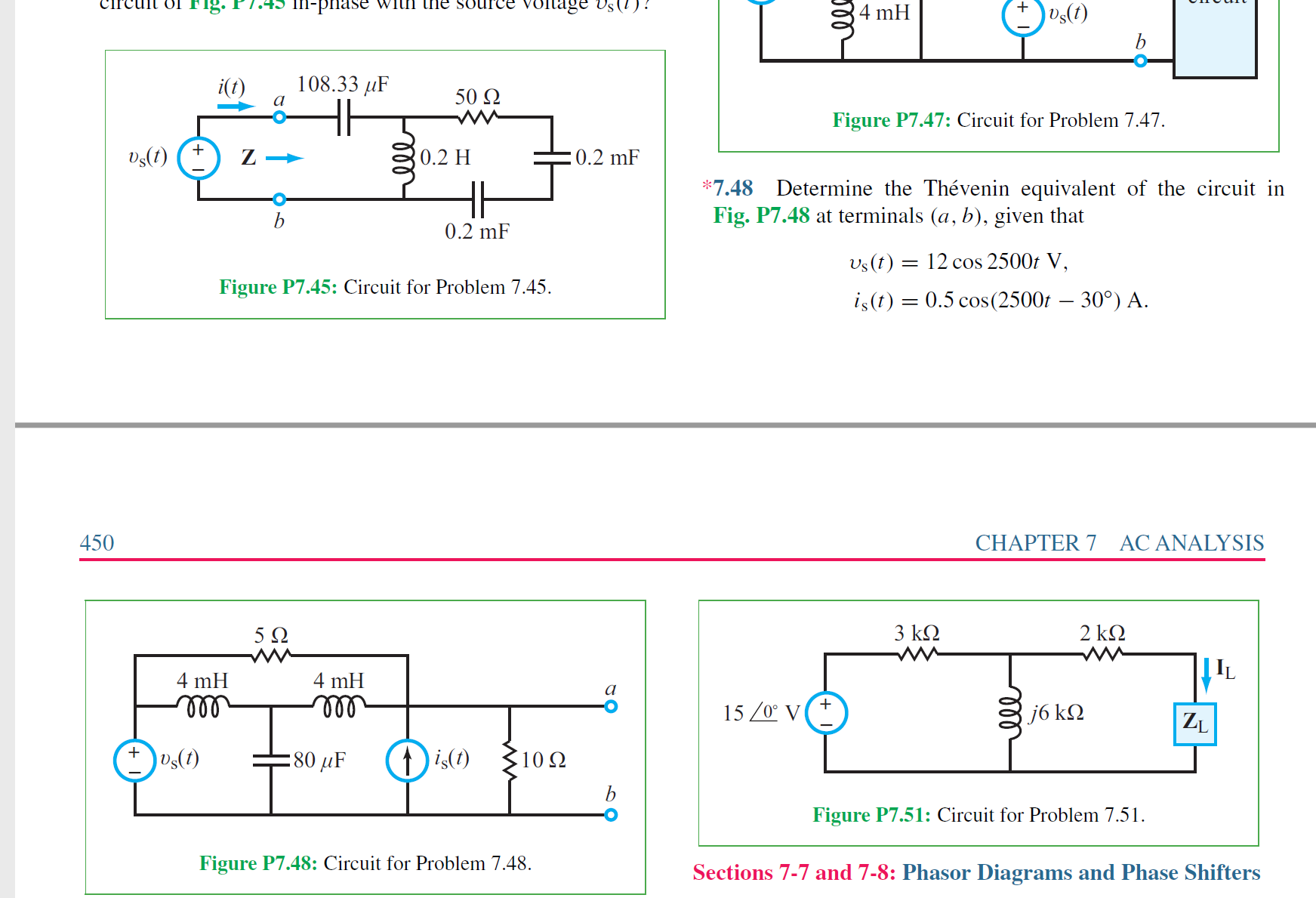# Circuit olrig. f'ce oltage Vs(1)! .45 M-p1ase withi lfe su (1)sa b 4 mH 108.33 iF i(t) 50 Ω Figure P7.47: Circuit for Problem 7.47 Ds(t) 0.2 H 0.2 mF 7.48 Determine the Thévenin equivalent of the circuit in Fig. P7.48 at terminals (a, b), given that b 0.2 mF Us(t)12 cos 2500t V Figure P7.45: Circuit for Problem 7.45 0.5 cos(2500r - 30°) A. is(t) 450 CHAPTER 7 AC ANALYSIS 2 kΩ 3 k2 5 Q W | IL 4 mH 4 mH 15/0 V j6 k ZL )Us(t) is) :80 μF 10Ω b Figure P7.51: Circuit for Problem 7.51 Figure P7.48: Circuit for Problem 7.48. Sections 7-7 and 7-8: Phasor Diagrams and Phase Shifters 000

Question

Determine the Th´evenin equivalent of the circuit in
Fig. P7.48 at terminals (a, b), given that
υs(t) = 12 cos 2500t V,
is(t) = 0.5 cos(2500t − 30◦
) A.help_outlineImage TranscriptioncloseCircuit olrig. f'ce oltage Vs(1)! .45 M-p1ase withi lfe su (1)sa b 4 mH 108.33 iF i(t) 50 Ω Figure P7.47: Circuit for Problem 7.47 Ds(t) 0.2 H 0.2 mF 7.48 Determine the Thévenin equivalent of the circuit in Fig. P7.48 at terminals (a, b), given that b 0.2 mF Us(t)12 cos 2500t V Figure P7.45: Circuit for Problem 7.45 0.5 cos(2500r - 30°) A. is(t) 450 CHAPTER 7 AC ANALYSIS 2 kΩ 3 k2 5 Q W | IL 4 mH 4 mH 15/0 V j6 k ZL )Us(t) is) :80 μF 10Ω b Figure P7.51: Circuit for Problem 7.51 Figure P7.48: Circuit for Problem 7.48. Sections 7-7 and 7-8: Phasor Diagrams and Phase Shifters 000 fullscreen